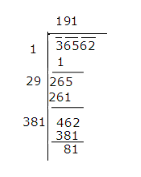# 36562 plants are to be planted in a garden in such a way that each row

36562 plants are to be planted in a garden in such a way that each row contains as many plants as the number of rows. How many plants would be left out in this arrangement?

It is given that 36562 plants are to be planted.
Number of rows = Number of plants in each row
The number of plants which will be left out in this arrangement has to be calculated. That is, the number which should be subtracted from 36562 to make it a perfect square has to be calculated.
The square root of 36562 can be calculated by long division method as follows.∴ Number of plants left = 81